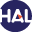Home page > 17. Volume 11 (2014) > An entropy preserving MOOD scheme for the Euler equations

# An entropy preserving MOOD scheme for the Euler equations

Tuesday 28 January 2014,

Abstract The present work concerns the derivation of entropy stability properties to be satisfied by high-order accurate finite volume methods. Such a sta- bility turns out to be crucial when approximating the weak solutions of hyperbolic systems of conservation laws. In fact, several recent works pro- pose some kind of discrete entropy inequalities associated to high-order schemes. However, these entropy preserving schemes do not seem relevant to impose that the converged solution (in the sense of the Lax-Wendroff Theorem) satisfies the required entropy inequalities. We illustrate such a failure by exhibiting numerical schemes that, from one hand, satisfy en- tropy stability and, from the other hand, do not prevent numerical blow- up. Here, we recall the expected high-order discrete entropy inequalities to be certain that the approximate solution converges to an entropy solution. Equipped with these sufficient numerical entropy stability, we propose to extend the recently introduced high-order MOOD scheme to satisfy the required high-order entropy inequalities. In fact, the MOOD approach is based on an a posteriori estimation and it seems impossible to impose a posteriori the whole set of discrete entropy inequalities. We solve this dif- ficulty by considering a finite volume scheme, which involves (at least one) discrete entropy inequalities with a numerical transport property. From one selected numerical transport discrete entropy inequality, we establish that all the needed discrete entropy inequalities are satisfied. Arguing this specific numerical transport entropy, we derive the expected a posteriori entropy condition to get an entropy preserving high-order MOOD scheme. Numerical experiments illustrate the relevance of the suggested numerical procedure.

Keywords : Euler equations, numerical approximation, finite volume methods, high-order approximation, discrete entropy inequalities.

Paper presented by: Professor Thierry GallouetVoir sur HAL : https://hal.archives-ouvertes.fr/hal-01115334